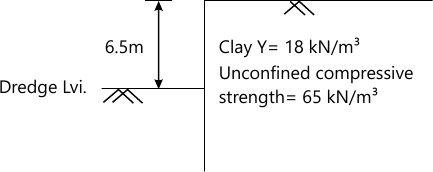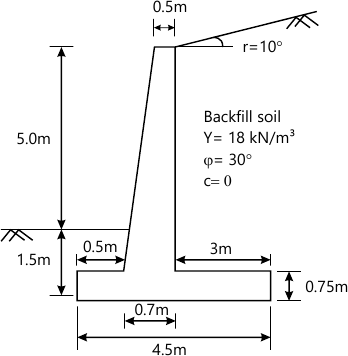MORE IN Geotechnical Engineering - 2
MU Civil Engineering (Semester 6)
Geotechnical Engineering - 2
May 2015
Total marks: --
Total time: --
INSTRUCTIONS
(1) Assume appropriate data and state your reasons
(2) Marks are given to the right of every question
(3) Draw neat diagrams wherever necessary

1 (a) Draw the free body diagram of forces for an embankment in c-ϕ soil with circular failure surface and define the notations used. Also determine the factor of safety with respect to cohesion for a submerged embankment 25 m high and having a slope of 40°. The properties of soil are ϕ=10°, c=40 kN/m2, γsat=19 kN/m3, Taylor's stability number, Sn=0.097.
5 M
1 (b) An unsupported excavation is to be made in soil having ϕ=12°, c=19 kN/m2, γ=19 kN/m3. What is the maximum depth of unsupported excavation in soil? And draw the active pressure distribution diagram.
5 M
1 (c) Considering Coulomb's theory of lateral earth pressure, draw and define the forces acting on the cohesionless backfill at failure under active state behind a retaining wall. Also, why are retaining walls usually designed for active earth pressure?
5 M
1 (d) Explain the basic difference in the theoretical bearing capacity computation of shallow and deep foundations.
5 M
1 (e) Define allowable bearing capacity of soil and what is the effect of increase in width and depth of a footing on the bearing capacity and settlement behaviour of footing resting on i) sand and ii) clay?
5 M
1 (f) In what respect does the design of braced cuts differ from that of a retaining wall and what are apparent earth pressure diagrams used in the design of braced cuts?
5 M

2 (a) Derive an expression for factor of safety of an infinite slope in a c-ϕ soil when there is a steady seepage parallel to the slope.
7 M
2 (b) A deep cut of 12 m depth is made soil for the construction of a road. The properties of soil are: cohesion=30 kN/m2, angle of internal friction =15° and a unit weight=20 kN/m3. The slope angle of the cut is 35°. Consider a trial slip circle of radius 20 m passing through the toe and cutting the top ground surface at a distance 5 m from top edge. Determine the factor of safety with respect to cohesion for the given trial slip circle by friction circle method. Assume factor of safety w.r.t friction as 1.5.
8 M
2 (c) Critically compare Rankine's theory with the Coulomb'd lateral earth pressure theory.
5 M

3 (a) Explain Culmann's method for the determination of active earth pressure on retaining wall considering the effect of line load on backfill.
8 M
3 (b) A 7 , retaining wall with a smooth vertical back face has a stratified back fill and a surcharge load of 10 kpa. The properties of soil are as follows up to 3.5 m height from top: unit weight=15 kN/m3, angle of shearing resistance =10° and cohesion=10 kpa. Draw the lateral active earth pressure diagram and estimate the resultant thrust on the wall and its position.
8 M
3 (c) What are the different types of conduits and the factor that affect the load on a conduit?
4 M

4 (a) For the cantilever sheet pile wall shown in Figure 1, compute the embedment depth below the dredge line.10 M
4 (b) Differentiate between general, local and punching shear failure of shallow foundations.
5 M
4 (c) Describe how the bearing capacity of soil for shadow foundation can be determined from standard penetration test.
5 M

5 (a) Check the stability of the wall shown in Figure 2. Unit weight of concrete=24 kN/m3.Foundation sail: Y = 19kN/m3, Ï• = 20°, C=40 kpa, &=23°
Safe bearing capacity = 500 kpa
10 M
5 (b) A circular foundation of 2.5 m diameter carries a load of 2500 kN. The soil has following properties :γ=19kN/m3, Φ =30°, c=4kN/m2, Nc=37.2, Nq=22.5, Nγ=19.7. Using Terzaghi's theory,
(a) Find the depth at which the foundation should be loaded to provide a factor of safety of 3.
(b) What is the depth of location of foundation if there exists a water table close to the ground surface?
10 M

6 (a) Explain the internal stability of mechanically stabilized retaining wall.
5 M
6 (b) Explain the method of computation of settlement of pile groups in clayey soil.
5 M
6 (c) A precast concrete pile of diameter 450 mm is driven into stiff clay. The unconfined compressive strength of the clay is 200 kN/m2. Determine the length of pile required to carry a safe load of 400 kN with factor of safety=2.5. Assume adhesion factor=0.55.
5 M
6 (d) Following data was obtained in a vertical pile load test on a 300 m diameter pile. Plot the load-settlement curve and determine the allowable load as per IS code.
 Load (kN) 50 100 200 300 400 500 600 Settlement (mm) 2.5 4 9.5 16.5 27 40.5 61
5 M

More question papers from Geotechnical Engineering - 2